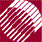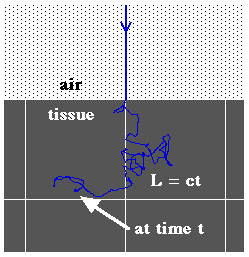ECE532 Biomedical Optics © 1998 Steven L. Jacques, Scott A. Prahl Oregon Graduate Institute

# Time-resolved Monte Carlo

## Introduction

We are already familiar with the simple Beer's Law description of photon survival in an absorbing medium:

survival = exp(-µaL)
µa
absorption coefficient [cm-1]
L
pathlength of photon travel [cm]

In a scattering medium, the photon's path is not a straight line, but Beer's law still holds. Regardless of how tortuous the path, the pathlength is given by:

L=ct
Pathlength
c
the speed of light in the medium (c = co/n)
t
time [s]At any point in time, one can calculate the probability of photon survival by exp(-µaL) = exp(-µact).

NextMonte Carlo